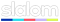# George Kosmidis

Microsoft MVP | Speaks of Azure & .NET | Founder of Munich .NET
Building tomorrow @# C#: Basic Combinatorics

by George Kosmidis / Published 10 years ago, modified 3 years and 3 months ago

Basic combinatorics in C# is a straight forward task for relatively small numbers like (100!). This is a helper class I wrote when I was asked to write an online lottery system.

Keep in mind though, that for bigger numbers (above 100!) you will need Arbitrary-precision arithmetic.

Here is the code and please, if someone knows a faster way or has some suggestions let me now!

``````public class BasicCombinatorics
{

///
/// One object in each position,
/// With elementary counting,
/// With repetitions,
/// With grouping of same objects
///
/// Number of objects
/// Groups of same objects
/// Number of permutations with groups of same objects
public double PermutationsSimilarGroups(int n, List ni)
{
var p = 1.0d;
foreach (var i in ni)
p *= Factorial(i);

return p / Factorial(n);
}

///
/// One object in each position,
/// With elementary counting,
/// With repetitions,
/// No groups of same objects
///
/// Number of objects
/// Number of positions
/// Number of provisions with repetition of n per k
public double ProvisionsReps(int n, int k)
{
return Math.Pow(n, k);
}

///
/// One object in each position,
/// With elementary counting,
/// No repetitions
///
/// Number of objects
/// Number of positions
/// Number of simple provisions
public double SimpleProvisions(int n, int k)
{
return Factorial(n) / Factorial(n - k);
}

///
/// One object in each position,
/// No elementary counting,
/// With repetitions
///
/// Number of objects
/// Number of positions
/// Number of combinations
public double CombinationsΡeps(int n, int k)
{
return Factorial(n + k - 1) / (Factorial(k) * Factorial(n - 1));
}

///
/// One object in each position,
/// No elementary counting,
/// No repetitions
///
/// Number of objects
/// Number of positions
/// Number of simple combinations
public double SimpleCombinations(int n, int k)
{
return Factorial(n) / (Factorial(k) * Factorial(n - k));
}

///
/// Many object in each position,
/// With same objects
///
/// Number of objects
/// Number of positions
/// Number of combinations of same objects
public double SameObjectsInSlots(int n, int m)
{
return Factorial(m + n - 1) / (Factorial(n) * Factorial(m - 1));
}

///
/// Many object in each position,
/// No same objects,
/// With distinguished objects
///
/// Number of objects
/// Number of positions
/// Number of combinations of distinguished objects
public double DifObjectsInSlotsBySeries(int n, int m)
{
return Factorial(m + n - 1) / Factorial(m - 1);
}

///
/// Many object in each position,
/// No same objects,
/// No distinguished objects
///
/// Number of objects
/// Number of positions
/// Number of combinations
public double DifObjectsInSlots(int n, int m)
{
return Math.Pow(m, n);
}

///
/// Factorial of a number
///
/// A number for factorial (n!)
/// n!
public double Factorial(int n)
{
double result = 1;
for (int i = n; i >= 1; i--)
result *= i;
return result;
}

///
/// Sum of a number (Σn)
///
/// A number for sum (Σn)
/// Σn
public double Sum(int n)
{
double result = 0;
for (int i = n; i >= 1; i--)
result += i;
return result;
}
}``````